Next: The query likelihood model Up: Language models Previous: Types of language models   Contents   Index

## Multinomial distributions over words

Under the unigram language model the order of words is irrelevant, and so such models are often called bag of words'' models, as discussed in Chapter 6 (page 6.2 ). Even though there is no conditioning on preceding context, this model nevertheless still gives the probability of a particular ordering of terms. However, any other ordering of this bag of terms will have the same probability. So, really, we have a multinomial distribution over words. So long as we stick to unigram models, the language model name and motivation could be viewed as historical rather than necessary. We could instead just refer to the model as a multinomial model. From this perspective, the equations presented above do not present the multinomial probability of a bag of words, since they do not sum over all possible orderings of those words, as is done by the multinomial coefficient (the first term on the right-hand side) in the standard presentation of a multinomial model: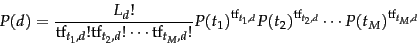(97)

Here,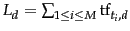is the length of document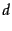,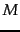is the size of the term vocabulary, and the products are now over the terms in the vocabulary, not the positions in the document. However, just as with STOP probabilities, in practice we can also leave out the multinomial coefficient in our calculations, since, for a particular bag of words, it will be a constant, and so it has no effect on the likelihood ratio of two different models generating a particular bag of words. Multinomial distributions also appear in Section 13.2 (page).

The fundamental problem in designing language models is that we do not know what exactly we should use as the model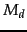. However, we do generally have a sample of text that is representative of that model. This problem makes a lot of sense in the original, primary uses of language models. For example, in speech recognition, we have a training sample of (spoken) text. But we have to expect that, in the future, users will use different words and in different sequences, which we have never observed before, and so the model has to generalize beyond the observed data to allow unknown words and sequences. This interpretation is not so clear in the IR case, where a document is finite and usually fixed. The strategy we adopt in IR is as follows. We pretend that the documentis only a representative sample of text drawn from a model distribution, treating it like a fine-grained topic. We then estimate a language model from this sample, and use that model to calculate the probability of observing any word sequence, and, finally, we rank documents according to their probability of generating the query.

Exercises.

• Including stop probabilities in the calculation, what will the sum of the probability estimates of all strings in the language of length 1 be? Assume that you generate a word and then decide whether to stop or not (i.e., the null string is not part of the language).

• If the stop probability is omitted from calculations, what will the sum of the scores assigned to strings in the language of length 1 be?

• What is the likelihood ratio of the document according to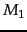and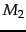in m1m2compare?

• No explicit STOP probability appeared in m1m2compare. Assuming that the STOP probability of each model is 0.1, does this change the likelihood ratio of a document according to the two models?

• How might a language model be used in a spelling correction system? In particular, consider the case of context-sensitive spelling correction, and correcting incorrect usages of words, such as their in Are you their? (See Section 3.5 (page) for pointers to some literature on this topic.)Next: The query likelihood model Up: Language models Previous: Types of language models   Contents   Index